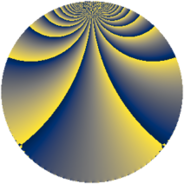# Properties

 Label 2304.3.nLevel $2304$ Weight $3$ Character orbit 2304.n Rep. character $\chi_{2304}(641,\cdot)$ Character field $\Q(\zeta_{6})$ Dimension $376$ Sturm bound $1152$

# Related objects

## Defining parameters

 Level: $$N$$ $$=$$ $$2304 = 2^{8} \cdot 3^{2}$$ Weight: $$k$$ $$=$$ $$3$$ Character orbit: $$[\chi]$$ $$=$$ 2304.n (of order $$6$$ and degree $$2$$) Character conductor: $$\operatorname{cond}(\chi)$$ $$=$$ $$72$$ Character field: $$\Q(\zeta_{6})$$ Sturm bound: $$1152$$

## Dimensions

The following table gives the dimensions of various subspaces of $$M_{3}(2304, [\chi])$$.

Total New Old
Modular forms 1584 392 1192
Cusp forms 1488 376 1112
Eisenstein series 96 16 80

## Trace form

 $$376q + 8q^{9} + O(q^{10})$$ $$376q + 8q^{9} - 856q^{25} - 44q^{33} + 12q^{41} - 1152q^{49} + 80q^{57} - 12q^{65} + 16q^{73} - 8q^{81} - 4q^{97} + O(q^{100})$$

## Decomposition of $$S_{3}^{\mathrm{new}}(2304, [\chi])$$ into newform subspaces

The newforms in this space have not yet been added to the LMFDB.

## Decomposition of $$S_{3}^{\mathrm{old}}(2304, [\chi])$$ into lower level spaces

$$S_{3}^{\mathrm{old}}(2304, [\chi]) \cong$$ $$S_{3}^{\mathrm{new}}(72, [\chi])$$$$^{\oplus 6}$$$$\oplus$$$$S_{3}^{\mathrm{new}}(288, [\chi])$$$$^{\oplus 4}$$$$\oplus$$$$S_{3}^{\mathrm{new}}(576, [\chi])$$$$^{\oplus 3}$$$$\oplus$$$$S_{3}^{\mathrm{new}}(1152, [\chi])$$$$^{\oplus 2}$$